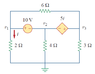# Circuit Analysis

• Engineering
• Dunder

## Homework Statement

Find v1, v2, v3
Must be done with nodal analysis

I=V/R

## The Attempt at a Solution

So i started by saying i1 = v2/4, i = v1/2, i3 = v3/3
i + i1 + i2 = 0
v3-v2=51
v1-v2=0

I am not really sure to what to do from here, i can't seem to come across the right solution, the answer given in the book is v1=3.043, v2=-6.956, v3=0.6522

Any help would be much appreciated

#### Attachments

•c1.PNG
4.2 KB · Views: 423

## Homework Statement

Find v1, v2, v3
Must be done with nodal analysis

I=V/R

## The Attempt at a Solution

So i started by saying i1 = v2/4, i = v1/2, i3 = v3/3
i + i1 + i2 = 0
v3-v2=51
v1-v2=0

I am not really sure to what to do from here, i can't seem to come across the right solution, the answer given in the book is v1=3.043, v2=-6.956, v3=0.6522

Any help would be much appreciated

The problem says to use nodal analysis. You haven't written any complete nodal equations.

Hint: The nodes designated by V1, V2, and V3 are all separated by voltage sources. This implies that you can treat all three nodes as one supernode. Write the nodal equation for the supernode by referencing v1, and note that i = v1/2Ω. (v2 = v1 - 10V; v3 = v1 - 10V + 5i).

So is my nodal equation with the super node i + i1 + i2 = 0 ?
The branch including the 6 ohm resister is negligible since it theoretically exits the node and enters it as well?
Using this, I ended up solving for v1, but ended up finding v1 = 0, so I must be going in the wrong direction

So is my nodal equation with the super node i + i1 + i2 = 0 ?
The branch including the 6 ohm resister is negligible since it theoretically exits the node and enters it as well?
That is true.
Using this, I ended up solving for v1, but ended up finding v1 = 0, so I must be going in the wrong direction

Node equations involve the node voltages and the resistances connected between nodes (including the reference, or "ground" node). You shouldn't need to designate any particular current as "i1", or "i2", etc. The exception here is that the current given the label "i" is important for the dependent voltage source. You should be able to replace it with a node branch current expression (v1/2).

Okay thank you, I worked to the right answer. Thank you again